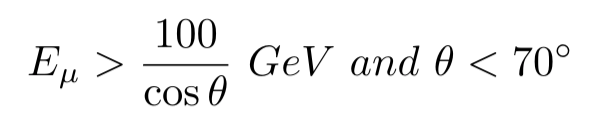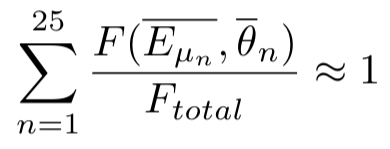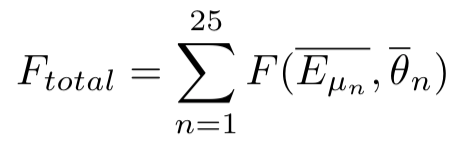# Analytical cosmic ray muon source sampling

Dear David,

I have been advancing in my work and now I am trying to work with cosmic rays at the surface of the earth, more specifically, muons.

I have a approximation formula for muons at the surface, but because of the forum rules I think I can’t post the original material. The conditions for the approximation to work are as follow:So my Probability Density Function would be:

Where A is the normalization factor.
After doing the integration A=1/0.0000112422

Now I need to calculate the Cumulative Distribution Function:

Where \xi is a random number from an uniform distribution [0,1)

After resolving my integral I had a hard time trying to find the inverse CDF function, so I used the Newton-Raphson Method:

With x_0=0.5D0

Finally, I did 4 beamspots because of symmetry to complete my distribution.

Could you check If I am doing any steps wrong and check my FLAIR output plot to make sure that indeed makes sense?

I attach my files. I don’t know if I cant attach my Mathematica output calculation because of the rules.
muonsatsurface.f (7.4 KB) muonsatsurface.flair (3.2 KB) muonsatsurface.inp (1.2 KB)

Best Regards,
Carlos

Dear Carlos,

I’m not an expert on statistics, so maybe I’m completely wrong.

I think you made an error, when you defined your CDF. You should not integrate it on the whole energy, but

F(E_\mu, \theta) = \int_{0}^{\theta} \int_{\frac{100}{cos \theta'}}^{E_\mu} f(E_\mu',\theta') dE_\mu' d\theta'

But, I don’t know who you can get the inverse CDF from this with two separate random numbers.

One solution to overcome this analytical problem, with discretization. You can set up a binning in energy and angle. Then using these you can create a table with the following 5 columns: E_\mu min, E_\mu max, \theta min, \theta max, and the calculated value based on the average energy and angle of the bin as relative “probabilities”.

Then you can use a random number to select a line based on the probabilities, and finally you can sample an exact energy and angle value based bin boundaries.

Cheers,
David

Dear David,

I am not sure if I understand what you are saying, please let me know if I am mistaken.

I defined the limits of E_\mu and \theta

I integrated my function using those limits: F_{total} =

I then made a .txt file with the following 6 columns:

Where

Where \Delta_E and \Delta_{\theta} are the bin width for muon energy and angle respectively.
ThenHere I have divided the Energy and Angle variables into 25 bins (n=25) for simplicity.

I would like to know: is the fifth column that you refer as “probability” in your previous message my sixth column?

Best Regards,
Carlos
discretemuons.txt (3.7 KB)

Dear Carlos,

yes, that would be the general idea, and I don’t see any mistakes with it.

Only one thing to add. You need to calculate F_{total} as

F_{total} = \sum_{n=1}^{N} F(\overline{E_{{\mu}_n}},\overline{\theta_n})

and not as the actual integral, to get the total probability exactly 1.0.

Finally, your 5th and 6th columns are redundant. The calculation of F_{total} and the normalization, can be done automatically in the source routine. Furthermore, even the whole table can be generated automatically if you prefer. Then you can only supply the source routine the minimum and maximum values and number of bins for E_{\mu} and \theta.

Cheers,
David

Dear David,

I am not well versed in Fortran, what do you mean by “automatically”.

I did change my integral F_total to:And my rows from the .txt to:

And my total probability indeed goes to 1.0.

Now I would sample \xi from an uniform distribution, I then would compare the value of \xi with another variable which would be the “accumulated probability” to chose a row. Is this correct? I attach my source.f if you could take a look.

Also I don’t understand what do you mean by sampling using the energy and angle from the bin boundaries. If you could give me extra clarification on that I would appreciate it.

discretemuons.txt (3.1 KB) muonsatsurface.f (4.5 KB)
Best Regards,
Carlos

Dear Carlos,

by automatically, I mean instead of using excel (or something else) to create the table, you can calculate the energy / angle steps and “probabilities”.

Please have a look at the function sample_histogram_momentum_energy() in source_library.inc located in the <path_to_fluka>/include/ directory.

From line 600 you see:

• calculating the cumulative probability
• selecting an energy bin
• finally sampling within the bin uniformly

Two comment on your source routine:

1. You need to set up arrays to store each line of your data file. See: Fortran - Arrays - Tutorialspoint
2. You should make sure, that you only read once from your file, not on every call to the source routine. To achieve this, take a look hot the first_run variable is used in the mentioned function.

If something still not clear, please let me know.

Cheers,
David

Dear David,

From sample_histogram_momentum_energy(char,char):
I don’t quite understand how the variable scale_factor works. Is It being converted from a character to a double precision variable? If yes then couldnt I just define it as sample_histogram_momentum_energy(char,double) in my function?

I did heavily modify my code to get it closer to the function you told me to look at, but I am still using my 5 column .txt file.

I attach all my files, if you could take a look at them I would appreciate it.

discretemuons.txt (1.2 MB) muonsatsurface.f (6.3 KB) muonsatsurface.flair (3.0 KB) muonsatsurface.inp (1.2 KB)

Best Regards,
Carlos

Dear Carlos,

the scale_factor variable and the unit conversion functions were only implemented, to allow inexperienced users covert easily the energy unit of the source file.